capacitance you know how inductive reactance and capacitive reactance act to oppose the flow of current in an ac circuit. However, there is another factor, the resistance, which also opposes the flow of the current. Since in practice ac circuits containing reactance also contain resistance, the two combine to oppose the flow of current.">Custom Search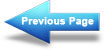Reactance, Impedance, and Power Relationships in AC Circuits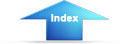Alternating CurrentOhms law for ACIMPEDANCE

From your study of inductance and capacitance you know how inductive reactance and capacitive reactance act to oppose the flow of current in an ac circuit. However, there is another factor, the resistance, which also opposes the flow of the current. Since in practice ac circuits containing reactance also contain resistance, the two combine to oppose the flow of current. This combined opposition by the resistance and the reactance is called the IMPEDANCE, and is represented by the symbol Z.

Since the values of resistance and reactance are both given in ohms, it might at first seem possible to determine the value of the impedance by simply adding them together. It cannot be done so easily, however. You know that in an ac circuit which contains only resistance, the current and the voltage will be in step (that is, in phase), and will reach their maximum values at the same instant. You also know that in an ac circuit containing only reactance the current will either lead or lag the voltage by one-quarter of a cycle or 90 degrees. Therefore, the voltage in a purely reactive circuit will differ in phase by 90 degrees from that in a purely resistive circuit and for this reason reactance and resistance are rot combined by simply adding them.

When reactance and resistance are combined, the value of the impedance will be greater than either. It is also true that the current will not be in step with the voltage nor will it differ in phase by exactly 90 degrees from the voltage, but it will be somewhere between the in-step and the 90-degree out-of-step conditions. The larger the reactance compared with the resistance, the more nearly the phase difference will approach 90. The larger the resistance compared to the reactance, the more nearly the phase difference will approach zero degrees.

If the value of resistance and reactance cannot simply be added together to find the impedance, or Z, how is it determined? Because the current through a resistor is in step with the voltage across it and the current in a reactance differs by 90 degrees from the voltage across it, the two are at right angles to each other. They can therefore be combined by means of the same method used in the construction of a right-angle triangle.

Assume you want to find the impedance of a series combination of 8 ohms resistance and 5 ohms inductive reactance. Start by drawing a horizontal line, R, representing 8 ohms resistance, as the base of the triangle. Then, since the effect of the reactance is always at right angles, or 90 degrees, to that of the resistance, draw the line XL, representing 5 ohms inductive reactance, as the altitude of the triangle. This is shown in figure 4-5. Now, complete the hypotenuse (longest side) of the triangle. Then, the hypotenuse represents the impedance of the circuit.

Figure 4-5. - Vector diagram showing relationship of resistance, inductive reactance, and impedance in a series circuit.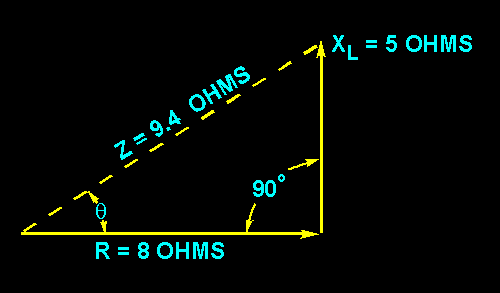One of the properties of a right triangle is: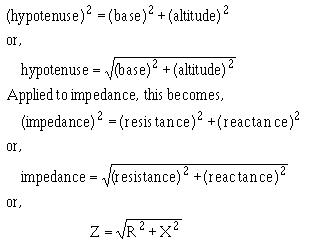Now suppose you apply this equation to check your results in the example given above.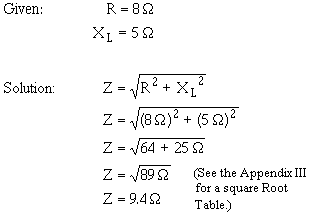When you have a capacitive reactance to deal with instead of inductive reactance as in the previous example, it is customary to draw the line representing the capacitive reactance in a downward direction. This is shown in figure 4-6. The line is drawn downward for capacitive reactance to indicate that it acts in a direction opposite to inductive reactance which is drawn upward. In a series circuit containing capacitive reactance the equation for finding the impedance becomes: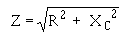Figure 4-6. - Vector diagram showing relationship of resistance, capacitive reactance, and impedance in a series circuit.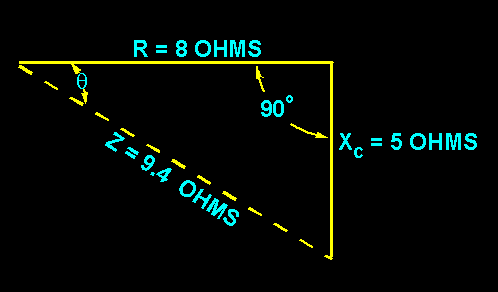In many series circuits you will find resistance combined with both inductive reactance and capacitive reactance. Since you know that the value of the reactance, X, is equal to the difference between the values of the inductive reactance, XL, and the capacitive reactance, XC, the equation for the impedance in a series circuit containing R, XL, and XC then becomes: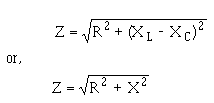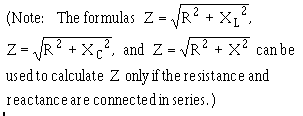In figure 4-7 you will see the method which may be used to determine the impedance in a series circuit consisting of resistance, inductance, and capacitance.

Figure 4-7. - Vector diagram showing relationship of resistance, reactance (capacitive and inductive), and impedance in a series circuit.Assume that 10 ohms inductive reactance and 20 ohms capacitive reactance are connected in series with 40 ohms resistance. Let the horizontal line represent the resistance R. The line drawn upward from the end of R, represents the inductive reactance, XL. Represent the capacitive reactance by a line drawn downward at right angles from the same end of R. The resultant of XL and XC is found by subtracting XL from XC. This resultant represents the value of X.

Thus: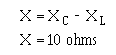The line, Z, will then represent the resultant of R and X. The value of Z can be calculated as follows: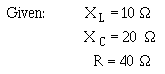Q.15 What term is given to total opposition to ac in a circuit?
Q.16 What formula is used to calculate the amount of this opposition in a series circuit?
Q.17 What is the value of Z in a series ac circuit where XL = 6 ohms, XC = 3 ohms, and R = 4 ohms?Integrated Publishing, Inc. - A (SDVOSB) Service Disabled Veteran Owned Small Business